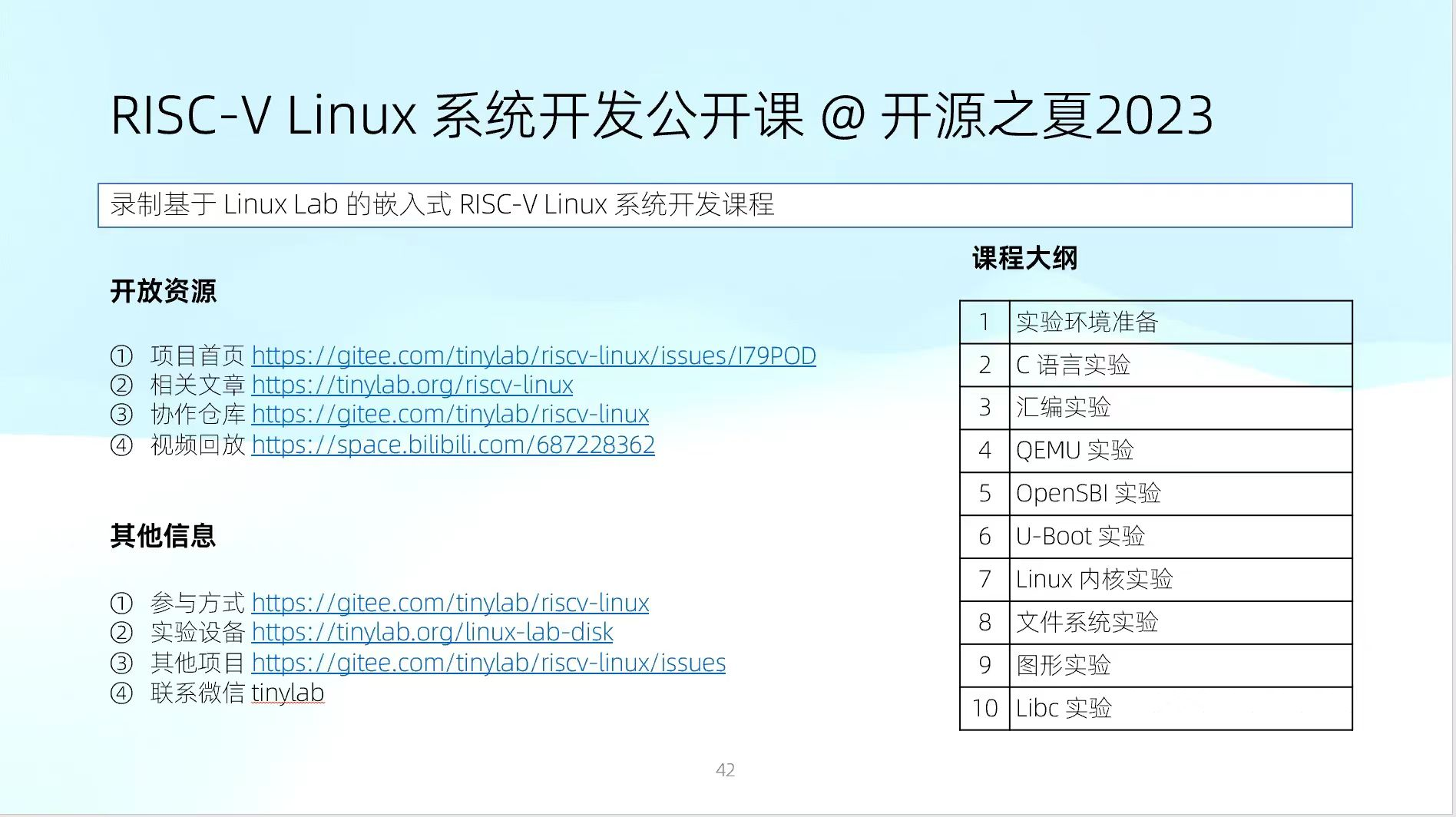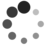# 11 个 Makefile 进阶用法

By Falcon of TinyLab.org Aug 01, 2019

## 立即赋值（:=）和延迟赋值（=）

• `:=`： 强制按先后顺序执行，立即赋值。
• `=`：赋值的结果会等到整个路径执行完再决定，后面的会覆盖前面的，延迟赋值。

``````\$ cat Makefile

a = foo
b1 := \$(a) bar
b2 = \$(a) bar
a = xyz

all:
@echo b1=\$(b1)
@echo b2=\$(b2)

\$ make
b1=foo bar
b2=xyz bar
``````

## 变量赋值 和 目标执行 之间的时序关系

``````\$ make a=b target
\$ make target a=b
\$ a=b make target
\$ export a=b && make target
``````

``````a = aaa

test1:
echo \$a

a = bbb

test2:
echo \$a
``````

``````\$ cat Makefile

a ?= aaa
b := \$(a)
c = \$(a)

a_origin = \$(origin a)
b_origin = \$(origin b)
c_origin = \$(origin c)

all:
@echo all:\$(a)
@echo all:\$(b)
@echo all:\$(c)
@echo all:\$(a_origin)
@echo all:\$(b_origin)
@echo all:\$(c_origin)

a = bbb
b := \$(a)
c = \$(a)

test1:
@echo test1:\$(a)
@echo test1:\$(b)
@echo test1:\$(c)
@echo test1:\$(a_origin)
@echo test1:\$(b_origin)
@echo test1:\$(c_origin)

a = ccc
b := \$(a)
c = \$(a)

test2:
@echo test2:\$(a)
@echo test2:\$(b)
@echo test2:\$(c)
@echo test2:\$(a_origin)
@echo test2:\$(b_origin)
@echo test2:\$(c_origin)

a = ddd
``````

``````\$ make
all:ddd
all:ccc
all:ddd
all:file
all:file
all:file
``````

``````a ?= aaa
b := \$(a)
c = \$(a)
``````

``````\$ make test1
test1:ddd
test1:ccc
test1:ddd
test1:file
test1:file
test1:file

\$ make test2
test2:ddd
test2:ccc
test2:ddd
test2:file
test2:file
test2:file
``````

``````\$ make a=fff
all:fff
all:fff
all:fff
all:command line
all:file
all:file
``````

``````\$ make b=fff
all:ddd
all:fff
all:ddd
all:file
all:command line
all:file
``````

``````\$ make c=fff
all:ddd
all:ccc
all:fff
all:file
all:file
all:command line
``````

``````\$ a=xxx make
all:ddd
all:ccc
all:ddd
all:file
all:file
all:file
``````

``````\$ a=xxx make -e
all:xxx
all:xxx
all:xxx
all:environment override
all:file
all:file
``````

``````\$ b=xxx make -e
all:ddd
all:xxx
all:ddd
all:file
all:environment override
all:file
``````

``````\$ export b=fff
\$ make -e
all:ddd
all:fff
all:ddd
all:file
all:environment override
all:file
``````

``````\$ b=xxx make -e b=yyy
all:ddd
all:yyy
all:ddd
all:file
all:command line
all:file
``````

• 所有变量语句的执行在 target 下的语句之前（每个 target 所属语句有一个 TAB 的缩进）。
• 变量 override 优先级：`command line > environment override > file`

``````\$ b=xxx make -e b=yyy all b=zzz test2 b=mmm
``````

## 如何获取 make 传递的所有参数和编译目标

``````\$ make test1 test2 test3 a=123 b=456
``````

`make` 后面的参数有两种类型，一种是命令行变量，一种是编译目标。

``````ifeq (\$(filter test1, \$(MAKECMDGOALS)), test1)
do something here
endif
``````

``````ifeq (\$(origin a), command line)
do something here
endif
``````

## Makefile 调试与跟踪方法一览

Debugging

``````\$ make --debug xxx
``````

Tracing

``````\$ make --trace xxx
``````

Logging

``````\$(info ...)
\$(warning ...)
\$(error ...)
``````

`error` 打印日志后立即退出，非常适合已经复现的错误。

Environment dumping

``````\$ make -p xxx > xxx.data.dump
``````

## Makefile 与 Shell 中的文件名处理差异

Makefile 中有类似 Shell 的 `dirname``basename` 命令，它们是：`dir`, `basename`, `notdir`，但是用法有差异，千万别弄混，下面来一个对比。

``````\$ cat Makefile
makefile:
@echo \$(dir \$a)
@echo \$(basename \$a)
@echo \$(notdir \$a)

shell:
@echo \$(shell dirname \$a)
@echo \$(shell basename \$a)

\$ make makefile a=/path/to/abc.efg.tgz
/path/to/
/path/to/abc.efg
abc.efg.tgz
\$ make shell a=/path/to/abc.efg.tgz
/path/to
abc.efg.tgz

\$ make makefile a=/path/to/
/path/to/
/path/to/

\$ make shell a=/path/to/
/path
to

\$ make makefile a=/path/to
/path/
/path/to
to
``````

/path/to/abc.efg.tgz取目录dirdirname

``````\$ cat Makefile
makefile:
@echo \$(patsubst %/,%,\$(dir \$(patsubst %/,%,\$a)))
@echo \$(notdir \$(patsubst %/,%,\$a))

shell:
@echo \$(shell dirname \$a)
@echo \$(shell basename \$a)

\$ make makefile a=/path/to/abc.efg.tgz
/path/to
abc.efg.tgz
\$ make shell a=/path/to/abc.efg.tgz
/path/to
abc.efg.tgz

\$ make shell a=/path/to/
/path
to
\$ make makefile a=/path/to/
/path
to
``````

## 在 Makefile 表达式中使用逗号和空格变量

``````empty :=
space := \$(empty) \$(empty)
comma := ,
``````

## 在 Makefile 中对软件版本号做差异化处理

Makefile 通常需要根据软件版本传递不同的参数，所以经常需要对软件版本号做比较。

``````LINUX_MAJOR_VER := \$(subst v,,\$(firstword \$(subst .,\$(space),\$(LINUX))))
LINUX_MINOR_VER := \$(subst v,,\$(word 2,\$(subst .,\$(space),\$(LINUX))))

ifeq (\$(shell [ \$(LINUX_MAJOR_VER) -lt 4 -o \$(LINUX_MAJOR_VER) -eq 4 -a \$(LINUX_MINOR_VER) -le 19 ]; echo \$\$?),0)
KERNEL_OLDDEFCONFIG := oldnoconfig
else
KERNEL_OLDDEFCONFIG := olddefconfig
endif
``````

``````KCONFIG_MAKEFILE := \$(KERNEL_SRC)/scripts/kconfig/Makefile
KERNEL_OLDDEFCONFIG := olddefconfig
ifeq (\$(KCONFIG_MAKEFILE), \$(wildcard \$(KCONFIG_MAKEFILE)))
ifneq (\$(shell grep olddefconfig -q \$(KCONFIG_MAKEFILE); echo \$\$?),0)
ifneq (\$(shell grep oldnoconfig -q \$(KCONFIG_MAKEFILE); echo \$\$?),0)
KERNEL_OLDDEFCONFIG := oldconfig
else
KERNEL_OLDDEFCONFIG := oldnoconfig
endif
endif
endif
``````

## 修改默认执行目标的简单方法

``````\$ make -p | grep makefile | grep -v ^#
.DEFAULT_GOAL := makefile
makefile:
``````

``````\$ make -p .DEFAULT_GOAL=shell a=/path/to/abc.efg.tgz | grep ^.DEFAULT_GOAL
.DEFAULT_GOAL = shell
``````

``````override .DEFAULT_GOAL := shell
``````

## 检查文件是否存在的两种方法

``````ifeq (\$(TEST_FILE), \$(wildcard \$(TEST_FILE)))
\$(info file exists)
endif

ifeq (\$(shell [ -f \$(TEST_FILE) ]; echo \$\$?), 0)
\$(info file exists)
endif
``````

## 如何类似普通程序一样把目标当变量使用

``````\$ cat Makefile

# Must put this at the end of Makefile, to make sure override the targets before here
# If the first argument is "xxx-run"...
first_target := \$(firstword \$(MAKECMDGOALS))
reserve_target := \$(first_target:-run=)

ifeq (\$(findstring -run,\$(first_target)),-run)
# use the rest as arguments for "run"
RUN_ARGS := \$(filter-out \$(reserve_target),\$(wordlist 2,\$(words \$(MAKECMDGOALS)),\$(MAKECMDGOALS)))
# ...and turn them into do-nothing targets
\$(eval \$(RUN_ARGS):;@:)
endif

test-run:
@echo \$(RUN_ARGS)

\$ make test-run test1 test2
``````

``````kernel-target1:
@make target1 -C /path/to/linux-src

kernel-target2:
@make target2 -C /path/to/linux-src
``````

``````kernel-run:
@make \$(arg1) -C /path/to/linux-src
``````

``````\$ make kernel-run target1
\$ make kernel-run target2
``````

``````\$ make kernel-run arg1=target1
\$ make kernel-run arg1=target2
``````

## 送您一枚免费体验卡